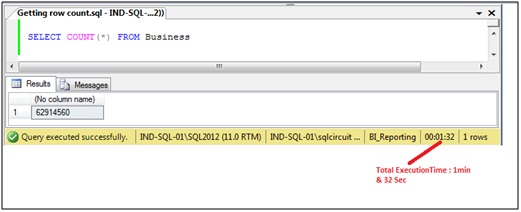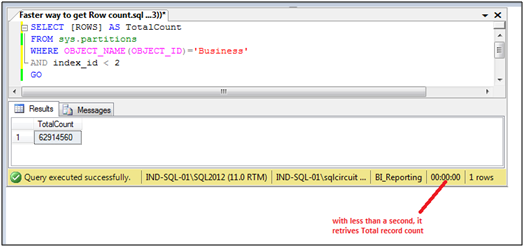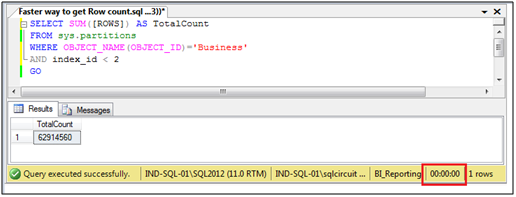# 1.     Background

The purpose of this article is to describe an efficient way of getting the row count of the table which is very fast as compare to COUNT (*) OR COUNT (Col1). Whenever we execute SELECT * FROM [TABLE] query, SQL Server performs table scan to get the total number of records in the table. If the table is bulky then it will take a long time to get the total record count. It will affect the performance of SQL stored procedure or function if we are using COUNT (*) function in them for bulky tables.

To resolve this issue, we are going to use sys.partitions system view which contains one row for each partition of all the tables and indexes except
Full-Text, Spatial, and XML type indexes.

# 2.     What is sys.partitions system view?

i.            Sys.partitions is a system view that provides information about partitions of tables/indexes whether table/indexes are explicitly partitioned or not.
ii.            Each row contains data for a partition including default partition.
iii.            Index_id: This column denotes type of index :
·         0 = heap
·         1 = clustered index
·         2 or greater = no clustered index
iv.            Partition_number:  This column contains partition number.
·         1 = Default Partition
·         > 1 = New partitions in sequential order
v.            ROWS:  This column contains no. of rows in the partition.

# 3.     Steps to get row count of table in faster way

i. Get the total record count of the table using COUNT (*) FUNCTION:COUNT(*) is taking approximately 1 minute and 32 seconds to retrieve total records count. Time taken by the COUNT (*) may be more and more if we have more records in the table. For a bulky table, COUNT (*) may affect your system performance too.

ii.    Now get the total record count of the table using sys.partitions system view:

SELECT [ROWS] AS TotalCount
FROM sys.partitions
AND index_id < 2
GOiii.      If your table is partitioned table then use the below T-SQL script to get total record count in faster way.

SELECT SUM([ROWS]) AS TotalCount
FROM sys.partitions